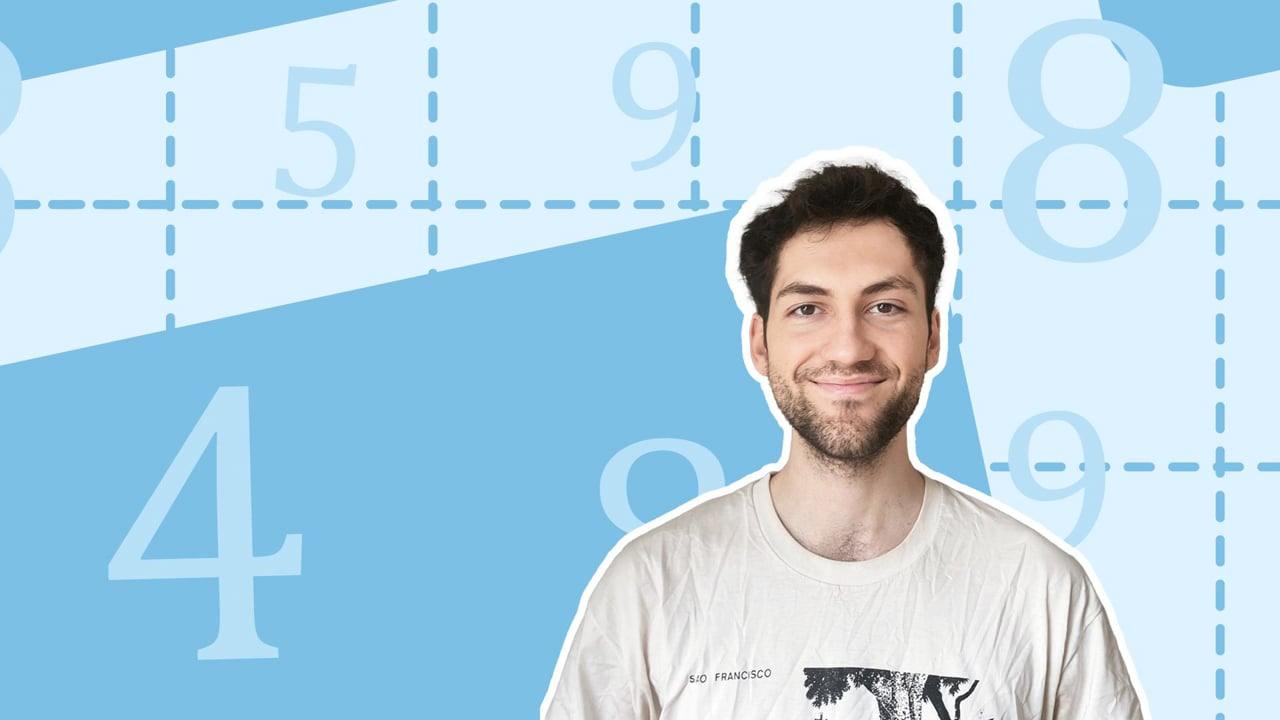Chapter overviewMaths

Number and place value

Multiplication and division

Fractions

Measurement

Geometry - properties of shapes

Geometry - position and direction

Statistics

Maths

# Understanding percentages0%

Summary

# Understanding percentages

## In a nutshell

The symbol that represents percent is $\%$​.  Per cent means "out of a hundred", and $100\%$ represents the whole amount.  Percentages can be written as fractions with a denominator of $100$​, or can be converted into decimals.

Curiosity: The word "percent" comes from the language Latin, which was spoken in ancient Rome. "Per" means "each" or "every", and "cent" means "one hundred". The word centimetre comes from the same root word - there are $100$ centimetres in a metre!

## Relating percentages and fractions

Percentages can be written as fractions by placing the percentage number over a denominator of $100$. The means that the percentage ($\%$) sign is effectively another way of writing $\dfrac{}{100}$.  Any percentage can be converted to a fraction with a denominator of $100.$​​

#### Procedure

 1 Recognise the percentage sign $(\%)$ and recall that this means "out of one hundred". 2 Write out a fraction with a denominator of $100.$​​ 3 Write the number in front of the percentage as the numerator of the fraction.

##### Example 1

Write $15\%$ as a fraction.

Recognise the $\%$​ and remember this relates to a fraction out of $100.$

$\%= \dfrac{}{100}$

Write the number in front of the percentage as the numerator of the fraction.

$15\%= \underline{\dfrac{15}{100}}$​​

Note: As you may have spotted, this fraction can be simplified, as $\dfrac{15}{100} = \dfrac{3}{20}$.  Make sure to fully simplify the fraction if the question asks you to.

##### Example 2

Are $40\%$ and $\dfrac{20}{50}$ equivalent?

Convert $40 \%$ into a fraction out of $100.$

$40 \% = \dfrac{40}{100}$​​

Expand the other fraction so that it has a denominator of $100$, to check whether the fractions are equivalent.

$\dfrac{20}{50}\times \dfrac{2}{2}=\dfrac{40}{100}$​​

Therefore:

$\underline{\text{Yes}},$​ $40 \%$​ and $\dfrac{20}{50}$are equivalent.

## Relating percentages and decimals

To change a percentage into a decimal, you divide by $100.$  To change a decimal into a percentage ($\%$), you apply the inverse operation and multiply by $100.$​​

##### Example 3

Change $42 \%$ into a decimal.

Divide the number in front of the percentage sign by $100$.

$42 \% \longrightarrow 42 \div 100 = 0.42$​​

Therefore:

$42\% = \underline{0.42}$​​

FAQs

• Question: What is a percentage?

Answer: The symbol that represents percent is %​. Per cent means "out of a hundred", and 100% represents the whole amount.

• Question: How are percentages and fractions related?

Answer: Percentages can be written as fractions by placing the percentage number over a denominator of 100. The means that the percentage (%) sign is effectively another way of writing /100.

• Question: How do I change a percentage into a decimal?

Answer: To change a percentage into a decimal, you divide the number in front of the percentage sign by 100.

Theory

Exercises